Lasso Selection (LASSO)

LASSO (least absolute shrinkage and selection operator) selection arises from a constrained form of ordinary least squares regression where the sum of the absolute values of the regression coefficients is constrained to be smaller than a specified parameter. More precisely let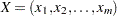denote the matrix of covariates and let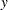denote the response, where the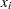s have been centered and scaled to have unit standard deviation and mean zero, andhas mean zero. Then for a given parameter, the LASSO regression coefficients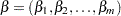are the solution to the constrained optimization problem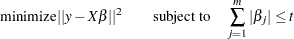Provided that the LASSO parameteris small enough, some of the regression coefficients will be exactly zero. Hence, you can view the LASSO as selecting a subset of the regression coefficients for each LASSO parameter. By increasing the LASSO parameter in discrete steps, you obtain a sequence of regression coefficients where the nonzero coefficients at each step correspond to selected parameters.

Early implementations (Tibshirani 1996) of LASSO selection used quadratic programming techniques to solve the constrained least squares problem for each LASSO parameter of interest. Later Osborne, Presnell, and Turlach (2000) developed a "homotopy method" that generates the LASSO solutions for all values of. Efron et al. (2004) derived a variant of their algorithm for least angle regression that can be used to obtain a sequence of LASSO solutions from which all other LASSO solutions can be obtained by linear interpolation. This algorithm for SELECTION=LASSO is used in PROC GLMSELECT. It can be viewed as a stepwise procedure with a single addition to or deletion from the set of nonzero regression coefficients at any step.

As with the other selection methods supported by PROC GLMSELECT, you can specify a criterion to choose among the models at each step of the LASSO algorithm with the CHOOSE= option. You can also specify a stopping criterion with the STOP= option. See the discussion in the section Forward Selection (FORWARD) for additional details. The model degrees of freedom PROC GLMSELECT uses at any step of the LASSO are simply the number of nonzero regression coefficients in the model at that step. Efron et al. (2004) cite empirical evidence for doing this but do not give any mathematical justification for this choice.

A modification of LASSO selection suggested in Efron et al. (2004) uses the LASSO algorithm to select the set of covariates in the model at any step, but uses ordinary least squares regression with just these covariates to obtain the regression coefficients. You can request this hybrid method by specifying the LSCOEFFS suboption of SELECTION=LASSO.